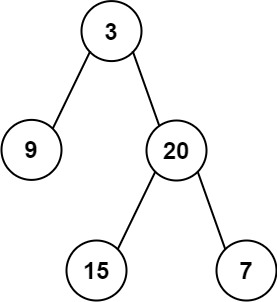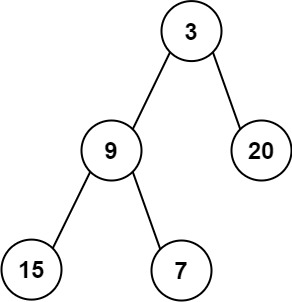# GeetCode Hub

Given the `root` of a binary tree, return the average value of the nodes on each level in the form of an array. Answers within `10-5` of the actual answer will be accepted.

Example 1:```Input: root = [3,9,20,null,15,7]
Output: [3.00000,14.50000,11.00000]
Explanation: The average value of nodes on level 0 is 3, on level 1 is 14.5, and on level 2 is 11.
Hence return [3, 14.5, 11].
```

Example 2:```Input: root = [3,9,20,15,7]
Output: [3.00000,14.50000,11.00000]
```

Constraints:

• The number of nodes in the tree is in the range `[1, 104]`.
• `-231 <= Node.val <= 231 - 1`

/** * Definition for a binary tree node. * public class TreeNode { * int val; * TreeNode left; * TreeNode right; * TreeNode() {} * TreeNode(int val) { this.val = val; } * TreeNode(int val, TreeNode left, TreeNode right) { * this.val = val; * this.left = left; * this.right = right; * } * } */ class Solution { public List<Double> averageOfLevels(TreeNode root) { } }